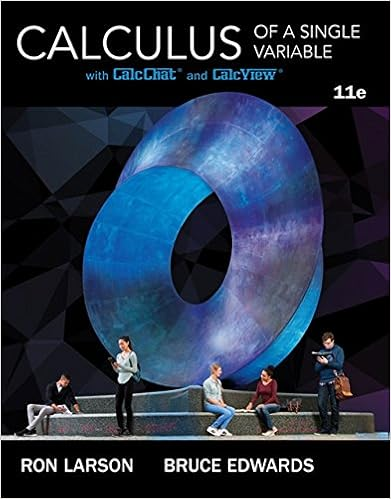# Midterm 1 Review.pdf - 1 What is the area under the graph...

• Test Prep
• 5

This preview shows page 1 - 5 out of 5 pages.

##### We have textbook solutions for you!
The document you are viewing contains questions related to this textbook.The document you are viewing contains questions related to this textbook.
Chapter 4 / Exercise 6
Calculus of a Single Variable
Edwards/LarsonExpert Verified
1 LARC Practice Midterm 1 1. What is the area under the graph when (a.) Set up an integral expression that describes the main question. (b.) Use the midpoint approximation to approximate the area under the curve when (c.) Set up the limit definition of integrals expression. (d.) Evaluate the Riemann sum set up in (c.). (e.) Check your answer by evaluating (a.)
##### We have textbook solutions for you!
The document you are viewing contains questions related to this textbook.The document you are viewing contains questions related to this textbook.
Chapter 4 / Exercise 6
Calculus of a Single Variable
Edwards/LarsonExpert Verified
2 2. is a function defined by the equation: Calculate the derivate 3. Calculate the following integrals:
•••